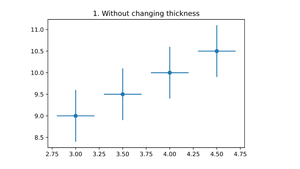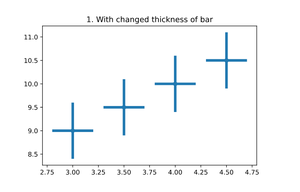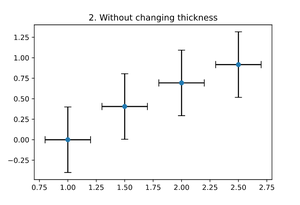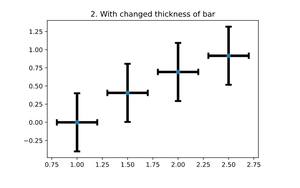GFG App
Open AppBrowser
Continue

# Change the error bar thickness in Matplotlib

Matplotlib is a Python library which is widely used by data analytics. Matplotlib.pyplot.errorbar()  is a pyplot module consisting of a function which provides a MATLAB like interface.

## Changing Error Bar Thickness

Before changing the thickness of error bar let us see what error bar is and how we can plot and use them.

Error Bar: Error bars are bars that we incorporate within our data conveying the unpredictability in reported measurements. In layman terms it displays graphical representations of the variableness of data and used on graphs to show the error measurement.

Syntax: matplotlib.pyplot.errorbar(x, y, yerr=None, xerr=None, fmt=”, ecolor=None, elinewidth=None, capsize=None, barsabove=False, lolims=False, uplims=False, xlolims=False, xuplims=False, errorevery=1, capthick=None, *, data=None, **kwargs)

Example 1:

## Python3

 `# importing necessary libraries` `import` `matplotlib.pyplot as plt`   `# example data` `x ``=` `np.arange(``3``, ``5``, ``0.5``)` `y ``=` `np.arange(``9``, ``11``, ``0.5``)`   `# plotting with default thickness of bar` `plt.title(``"1. Without changing thickness"``)` `plt.errorbar(x, y, xerr``=``0.2``, yerr``=``0.6``, fmt``=``'o'``)` `plt.show()`   `# plotting with changed thickness of bar` `plt.title(``"1. With changed thickness of bar"``)`   `# change elinewidth to change the thickness of bar` `plt.errorbar(x, y, xerr``=``0.2``, yerr``=``0.6``, fmt``=``'o'``, elinewidth``=``4``)` `plt.show()`

Output :Figure 1.1Figure 1.2

As we can see the difference in both the images. The elinewidth parameter takes the integer value and then increase the thickness of error bar.

Example 2:

## Python3

 `# importing necessary libraries` `import` `matplotlib.pyplot as plt`   `# example data` `x ``=` `np.arange(``1``, ``3``, ``0.5``)` `y ``=` `np.log(x)`   `# plotting with default thickness of bar` `plt.title(``"2. Without changing thickness"``)` `plt.errorbar(x, y, xerr``=``0.2``, yerr``=``0.4``, fmt``=``'o'``, ecolor``=``'black'``, capsize``=``5``)` `plt.show()`   `# plotting with changed thickness of bar` `# change elinewidth to change the thickness of bar` `plt.title(``"2. With changed thickness of bar"``)` `plt.errorbar(x, y, xerr``=``0.2``, yerr``=``0.4``, fmt``=``'o'``, elinewidth``=``4``,` `             ``ecolor``=``'black'``, capsize``=``5``, capthick``=``3``)`   `plt.show()`

Output :Figure 2.1Figure 2.2

In the above example we have applied to capsize as caps containing the complete information regarding how big the uncertainty is. If the bar is smaller, lower the std and lower the spread of data which means that data is concentrated around mean and if the bar is larger, larger the std and larger the spread of data.

My Personal Notes arrow_drop_up